## Introduction

Hybrid organic–inorganic perovskites1,2,3,4 recently surged to worldwide attention following the report of highly efficient solar cells based on methylammonium lead halides5,6,7,8. In fact, within less than 2 years of development, the photovoltaic energy-conversion efficiency of these devices increased from the initial 10.9% (ref. 5) to the current record of 17.9% (NREL certified) and 19.3% (uncertified)9. This impressive trend fuelled the expectation that perovskite photovoltaics may soon revolutionize the solar energy landscape.

Given the unusually long lifetimes of the photoexcited carriers10,11,12 and the relatively low optical gap13,14, it has been proposed that the perovskite layer may act both as the light absorber and as the electron and hole transporter15, similarly to bulk semiconductor solar cells. Within this scenario, it is expected that the energy-conversion efficiency can be increased by following the standard Schockley–Queisser analysis, that is by reducing the optical band gap to values near 1.3–1.4 eV (ref. 16).

Substantial efforts are currently underway to optimize these solar cells by modifying the metal–halide network or the organic cation13,14,17,18,19,20,21,22,23. A major challenge in this research area is that, on the experimental front, the structural, electronic and optical properties of metal-organic perovskites are very sensitive to processing conditions. Similarly, computational modelling studies appear sensitive to the level of theory and calculation details. This state of affairs suggests that it may be convenient to shift the focus from the investigation of a few prototypical perovskites to global searches relying on rational design approaches.

In this work we propose that, instead of modifying the metal–halide network as already conducted in refs 13, 14, it could be advantageous to start from MA-PbI3 (MA=CH3NH3) and modify its electronic and optical properties without altering the PbI3 network. This strategy would allow us to investigate potential improvements while retaining highly desirable features such as long carrier lifetimes10,12. In the following, using a combination of geometric arguments and first-principles calculations, we demonstrate that it should indeed be possible to modulate the optical gap of lead-iodide perovskites over almost an electronvolt by acting on the Pb–I–Pb bond angles in the PbI3 network. These angles, in turn, can be tailored using cations of different sizes, without altering the metal–halide chemistry.

## Results

### Platonic model of metal-halide perovskites

MA-PbI3 belongs to the family of perovskites of chemical formula ABX3. The simplest possible ABX3 perovskite consists of a high-symmetry cubic structure belonging to the space group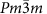, as shown in Fig. 1a (ref. 24). In this structure the halogen atoms X=I occupy the vertices of regular corner-sharing BX6 octahedra, while the divalent metal atoms B=Pb sit at the centres of the octahedra. The smallest volume enclosed by neighbouring octahedra defines a cuboctahedral cavity and hosts the monovalent cation A=CH3NH3. All the other possible perovskite structures can be imagined as obtained by rotating or distorting the BX6 octahedra, displacing the B metal atoms off-centre and rotating the A cations within the cuboctahedral cavity24.Figure 1: Platonic model of metal–halide perovskites.

Figure 1b shows the electronic band structure of MA-PbI3, which represents the starting point of our investigation. Here we see that the electronic states at the top of the valence bands are of halide p-character, while those at the bottom of the conduction band are mainly derived from the metal p states. At the same time the electronic states associated with the cation define bonding and antibonding bands arranged symmetrically around the band gap and located several electronvolts away from the band edges. An analysis of the electronic charge density shows that, as expected, the cation is singly ionized, and the PbI3 network is negatively charged25,26,27. These observations suggest that the electronic structure of these metal-organic perovskites may be described by removing the cation from the structural model and compensating the negative charge of the PbI3 network using a positive background. Figure 1b shows that the band structure obtained within this approximation is in good agreement with that from a complete calculation. The removal of the cation is especially useful since it eliminates the complications associated with the observed orientational disorder13,17,28,29. Another important observation can be made by inspecting X-ray diffraction data, as reported for example in ref. 17. In fact, from the structural parameters reported in that work we deduce that the Pb–I bond lengths are extremely regular, the largest relative deviation from the average value of 3.18 Å being 0.1%. Furthermore, the diagonals of the PbI6 octahedra are almost perpendicular to each other, the maximum deviation from right angles being of 4°. Therefore, to a good approximation the PbI6 octahedra may be considered as Platonic solids carrying the full Oh symmetry.

On the basis of these considerations we set to build a Platonic model of metal-halide perovskites, consisting of regular corner-sharing PbI6 octahedra and no cation, as shown in Fig. 1d. In order to construct our model we start from a single regular octahedron in an orthorhombic unit cell17, with one diagonal parallel to the c axis, and the four perpendicular edges parallel to the axes a and b. In the reference frame of the octahedron this diagonal and its perpendicular edges define the apical and equatorial directions, respectively. Within this model, the most general perovskite structure is obtained by three successive rotations around c, a and c again using the standard Euler angles30, as shown in Fig. 1c: the spinning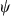around the apical direction, the tilt θ around the a axis and the precession φ around the c axis. After these rotations, the location and orientation of all the remaining octahedra in the orthorhombic unit cell are completely determined by the corner-sharing connectivity, the metal-halide bond length and the periodicity of the crystal structure. The details of this geometric construction are given in the Methods.

An immediate result of our construction is that the only way to ensure corner-sharing connectivity is by having a vanishing spinning angle. This leaves us with only two independent angular parameters, θ and φ, which refer to a spherical system of coordinates clamped on the crystallographic unit cell. It is convenient to make a change of variables to intrinsic angular parameters that are independent on the choice of the unit cell. To this end we express θ and φ in terms of the metal-halide–metal bond angles αa and αe, as indicated in Fig. 1d. αa denotes the Pb–I–Pb bond angles along the apical direction and αe those along the equatorial direction. The relation between these intrinsic angles and the Euler angles is given in the Methods.

At this point the atomic structure of the Platonic perovskite model is uniquely determined by the choice of two metal–halide–metal bond angles and the metal–halide bond length. Given that the effect of bond length variations on the electronic and optical properties of these systems has been studied in detail, both theoretically27 and experimentally13, our geometric analysis clearly directs attention to the role of the two inequivalent Pb–I–Pb angles.

### Band gap and metal–halide–metal bond angle

In Fig. 2 we show the band gap of the Platonic model calculated using density functional theory (DFT) as a function of the apical and equatorial bond angles αa and αe. For definiteness we set the Pb–I bond length to the average value measured in ref. 17. The incomplete coverage of the parameter space in Fig. 2 reflects the fact that only certain pairs of apical and equatorial angles are compatible with the corner-sharing connectivity, as discussed in the Methods. The extremal parameters of this map are, on the one side, αa=αe=180° (bottom left corner), corresponding to the most symmetric cubic perovskite structure with collinear Pb–I–Pb bonds. On the other side we have αa=αe=120° (top right corner), which we have been chosen by considering a limiting structure where the shortest distance between iodine atoms belonging to different octahedra matches the I2 bond length (αa=0 and αe=126°).Figure 2: Mapping the band gap landscape of metal-halide perovskites.

The variation of the angular coordinates in Fig. 2 clearly induces a substantial modulation of the band gap, from the top of the mid-infrared (1.1 eV) to the beginning of the visible spectrum (1.9 eV). This trend is in line with earlier calculations on simpler two-dimensional (2D) Sn-I perovskites for solution-processable electronics31.

The qualitative trend shown in Fig. 2 can be interpreted using elementary tight-binding arguments. If we consider for definiteness the bottom of the conduction band at Γ, which is most affected by bond angles, in the case of αa=αe=180° there are three degenerate electronic states derived from the 6p orbitals of Pb (without considering spin–orbit coupling for simplicity; this coupling is fully included in all our final results presented in Fig. 3b). It is intuitive that the energy of these states results mainly from ppσ bonding integrals since all the Pb-I bonds are collinear32. By moving away from the bottom left corner, the degeneracy is lifted as the bond integrals acquire ppπ components weighted by the angles αa and αe. Both components tend to raise the energy levels; therefore, the conduction band bottom results from the competition between the antibonding orbitals with the lowest energy along the apical or the equatorial direction. This is consistent with the approximate symmetry of the map in Fig. 2 with respect to the line corresponding to αa=αe. More importantly, this reasoning suggests that the band gap of the Platonic perovskite model is governed by the largest Pb–I–Pb bond angle in the metal–halide network, consistent with the trend in Fig. 2. This relation between band gap and bond angles holds unchanged when considering fully relativistic calculations including spin–orbit corrections. In fact, as shown in Supplementary Fig. 1, relativistic effects induce a large but slowly varying red shift of 0.8–1.1 eV across the family of compounds considered in this work. For the sake of completeness we also checked that excitonic effects play only a minor role, with exciton-binding energies never exceeding 80 meV as shown by Supplementary Fig. 2. Details on the calculation of exciton binding energies are given in the Methods, and the results are discussed in the Supplementary Note 1.Figure 3: Tuning the band gap of metal-halide perovskites via the steric size of the cation.

After taking into account the presence of the cation and the variations in the Pb-I bond lengths and Pb–I–Pb angles, the calculated band gaps deviate from the idealized predictions in Fig. 2 by 0.1–0.3 eV (see Fig. 3 and discussion below). Nonetheless, the general trend, which spans a range of up to 1 eV, is robust. This picture is also confirmed by relativistic GW calculations on a few selected compounds, as shown in Supplementary Fig. 4. The trend identified here suggests that in order to make low-gap perovskites for optimum photovoltaic efficiency we need to engineer structures with minimal octahedral tilt. On the other hand, in order to make large-gap perovskites for light-emitting diodes operating in the visible, we need to design structures with maximum tilt. The remaining question is how the tilt angle can be controlled.

### Controlling metal–halide–metal bond angles via the steric size of the cation

The Pb–I–Pb bond angles define the volume of the cuboctahedral cavity; therefore, it is intuitive that the use of larger cations may lead to values of αa and αe closer to straight angles33. In the search for such cations we perform DFT calculations for existing structures as well as for many hypothetical structures not considered hitherto, including full structural optimizations and spin–orbit interactions, in the presence of the cation (see Methods). In order to relate these structures and their properties to the predictions of the Platonic model, we consider the average values of the Pb–I–Pb bond angles and of the Pb–I bond length over a crystalline unit cell. These values are used as the coordinates needed for locating each structure on the map of Fig. 2.

Previously reported cations are methylammonium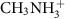(ref. 17), formamidinium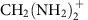(refs 13, 18), the alkali metal Cs+18 (synthesised) and ammonium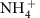(refs 34, 35, 36) (proposed). As shown in Fig. 2 all these cations cluster around the centre of the map; therefore, they are not expected to yield a significant modulation of the band gap. In line with this result their measured optical absorption onsets are very similar, within 0.25 eV (refs 13, 18).

In order to explore a wider portion of the map we consider four different families of cations generated from those above. The first family consists of secondary, tertiary and quaternary ammonium cations, namely di-, tri- and tetra-methylammonium. These are large molecules obtained by replacing hydrogen atoms bonded to the N atom with methyl groups. The second family is generated from ammonium by descending the pnictogen column in the periodic table, that is by substituting N for P, As or Sb. Members of this family include phosphonium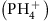, arsonium, stibonium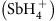, and similarly the methylammonium analogues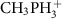,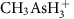and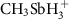. The third family of cations that we consider is obtained by replacing hydrogen in ammonium by halogen atoms, and possibly nitrogen by another pnictogen: in this group we have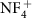,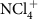,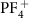, and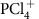. The fourth and final family simply consists of the alkali metals Li+, Na+, K+ and Rb+. In total, we consider 22 structures, including 18 hypothetical compounds not reported to date.

In order to quantify the steric size of each cation, we use the radius of the sphere that contains 95% of the DFT electron density. This choice ensures that the steric radii of the alkali metals are in agreement with their standard ionic radii37. For completeness the calculated steric sizes are reported in Supplementary Table 1. Figure 3 shows that the steric radii of the cations considered in this work span a wide range, from 0.7 Å (Li+) to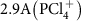.

Our structural optimizations indicate that for some of the large cations the three-dimensional (3D) perovskite network is significantly distorted. This is in line with previous studies showing that large molecular cations determine a reorganization of the 3D perovskite network into a 2D layered structure38,39. These large molecules include tertiary and quaternary methylammonium cations, as well as the tetrafluorideand the tetrachloride. As these structures depart substantially from the 3D metal–halide network considered in this work, a separate analysis would be required. Accordingly, we do not consider them further.

Figure 3a shows that the largest Pb–I–Pb angles correlate strongly with the steric size of the cation (Spearman correlation 88%), in line with our initial expectation. The newly proposed structures span a range of angles from 130° to 170°, thereby covering a much wider portion of the band gap map in Fig. 2 than presently possible. In addition, Fig. 3b shows that, in agreement with the predictions of the Platonic model, the band gap correlates strongly with the largest Pb–I–Pb angle in the unit cell (Spearman correlation 91%). For completeness in Fig. 3b we report the band gaps obtained from this model as well as those calculated using the fully optimized structures within fully relativistic DFT calculations. The scatter of the data, which is clearly visible in Fig. 3b, is mostly because of the variation of the Pb–I bond lengths in the metal–halide network. We calculated the correlation coefficient between band gap and bond lengths using the same data, and we obtained a very weak correlation (Spearman coefficient 9%). This test clearly indicates that the role of bond lengths represents a second-order effect, thereby providing further support to our Platonic model. Finally, Fig. 3c shows our main finding, namely that by suitably choosing the cation it is possible, at least in principle, to fine-tune the band gap almost continuously over a very wide range of photon energies.

Our analysis highlights several new promising structures, in particular perovskites with phosphonium, arsonium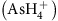and stibonium, all with band gaps ranging between 1.2 and 1.4 eV. These values fall in the middle of the range for optimum photovoltaic efficiency, and suggest that our new structures hold potential as novel solar cells materials. Besides the low gap, these perovskites are expected to exhibit higher mobilities than MA–PbI3. In fact, as shown in Supplementary Fig. 2a,b, the carrier-effective masses decrease towards larger bond angles, consistent with the concomitant reduction of the band gap. In addition to lower gaps and effective masses, large cations have the advantage that the filling of the cuboctahedral cavity should counter the known tendency of perovskite solar cells to degrade by water incorporation14.

In order to evaluate the practical feasibility of the hypothetical perovskites identified in this work we searched for possible dynamical instabilities in one of the structures with the smallest band gap, AsH4PbI3 (is less interesting because of safety concerns related to chlorides). This structure is especially interesting since the preparation could proceed through the corresponding iodide AsH4I (ref. 40), similarly to the case of methylammonium iodide CH3NH3I. As shown by Supplementary Fig. 3, the vibrational density of states calculated using density-functional perturbation theory41 does not exhibit any soft modes, thereby indicating that the 3D perovskite structure of AsH4PbI3 should be stable against distortions. More generally, in order to assess the stability of all the structures considered here, we move to a simple semi-empirical approach based on the Goldschmidt tolerance factor t (refs 33, 42) (see Methods for the definition of t). It is known empirically that 3D perovskites should form when the tolerance factor falls within a narrow range t=0.7–1.1 (refs 33, 42, 43, 44). Figure 4a shows that the tolerance factors derived from our calculated steric sizes falls within this range for most of the structures considered here. In particular, according to this criterion, all the cations up to Rb and K should be stable.Figure 4: Material stability and optical measurements.

In order to demonstrate the band gap tunability we attempted the synthesis of perovskites based on elemental cations, building on our experience with CsPbI3 (ref. 18) (see Methods). As deposited, we observed that CsPbI3 formed a pale phase with a wide bandgap. Upon annealing this structure turned into a dark phase with a band gap of 1.73 eV, which we identify as the 3D perovskite structure discussed in this work. The synthesis of films with cations smaller than Cs (Rb, K, Na and Li) yielded similar pale phases. However, upon annealing, some of the films degraded via sublimation before we could observe a dark phase as for CsPbI3, suggesting that 3D perovskites were not formed. This finding is in agreement with our analysis of the Goldschmidt tolerance factor shown in Fig. 4a. While the proposed 3D perovskites could possibly be synthesised using higher pressures or longer annealing runs at lower temperatures, here for the sake of simplicity we did not pursue this direction. Instead we considered an alternative approach and explored mixtures of cations, as suggested in ref. 45: if mixtures of Cs and smaller cations could gradually shift the bandgap towards the larger values, this would confirm our predicted tunabiliy via steric effects.

As a proof-of-concept we prepared perovskites with Rb/Cs cation mixtures, namely RbxCs1−xPbI3 with x=0, x=0.1 and x=0.2 (never hitherto reported). Optical characterization of these films, shown in Fig. 4b, indicates that the absorption edge blue shifts from 720 to 690 nm in going from Cs to Rb. This result is in excellent agreement with our calculations, which predict a corresponding blue shift of 40 nm.

Our findings are also in line with a recent study (which appeared while the present manuscript was under review) where the authors demonstrated band gap tunability via the mixing of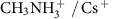mixtures46. Taken together, our present results and those of refs 13, 18, 47 confirm the tunability of the band gap via the steric size of the cation over almost half of our predicted range, thereby providing strong support to our theory.

Finally, our theory is based on very general considerations and its predictions are not linked to the underlying calculation methods. Therefore, it is expected that this model will carry similar predictive power across other families of metal–halide perovskites. More fundamentally, the ability to control the band gap via the steric size of the cation, as proposed in this work, may become a new paradigm in the solution deposition of solar cells, light-emitting diodes and photonic structures with layer-by-layer control of their optical properties.

Note added in proof. While this work was under peer review, a related work exploring the relation between the size of cations and the electronic and optical properties of metal-halide perovskites was submitted and published63. The key difference between the present work and that of ref. 63 is that we develop a predictive universal theory of band gap tuning via steric effects, and we perform experiments that confirm a posteriori our predictions.

## Methods

### Computational methods

All calculations were performed within the local density approximation to density functional theory48,49 using planewaves and pseudopotentials, as implemented in the Quantum ESPRESSO software package50. Only valence electrons were described explicitly, and the core–valence interaction was taken into account by means of ultrasoft pseudopotentials51,52 with nonlinear core correction53. For Pb and I we used fully relativistic ultrasoft pseudopotentials. In the case of Pb we included the 5d semicore states, and for Sb, Rb and Cs we used norm conserving pseudopotentials54. The electron wavefunctions and the charge density were expanded in planewave basis sets with kinetic energy cutoffs 40 and 200 Ry, respectively. Structural optimization was performed by sampling the Brillouin zone using uniform and unshifted 4 × 4 × 4 meshes. Electronic structure calculations were performed using a 6 × 6 × 6 Brillouin zone grid. All structural optimizations were initialized using the crystallographic data for MA–PbI3 reported in ref. 17. The initial coordinates of the molecular cations were chosen in such a way that the centre of mass is located in the middle of the cuboctahedral cavity, and the orientation of the four inequivalent cations in one unit cell follows the symmetry properties of the Pnma space group. Given the complexity of the total energy landscape of these systems, we checked the sensitivity of the structure with respect to the choice of the initial configuration in the case of NH4PbI3. This compound lies close to the stability limit (Fig. 4b), and can form quasi-1D structures in the presence of water55,56. In this case we performed structural optimizations using three additional initial configurations: (i) αa=αe=0, (ii) αa=0 and αe≠0 with octahedra in phase along the apical direction, (iii) same as in (ii) but with octahedra out of phase. The optimized structures are all 3D perovskites, with total energy differences within 6 meV per atom. The largest deviation from the band gap reported in Fig. 3c is of 0.2 eV, and reflects the differences in the bond angles, following the trend predicted by the Platonic model in Fig. 2.

In the case of MA–PbI3 it has been established that the spin–orbit interaction tends to reduce the scalar-relativistic band gap by ~1–1.1 eV (refs 20, 22). This is consistent with our results shown in Fig. 3b. On the other hand, GW quasiparticle corrections do increase the value of the band gap22. Given these compensating corrections, and the fact that the very small relativistic band gaps are likely to induce significant errors in the calculation of the screened Coulomb interaction W, it is sensible to take scalar-relativistic calculations as the most consistent and unambiguous way to capture trends and identify the most promising structures.

In order to check that the band gap trend obtained with DFT/LDA holds when using a higher level theory, we performed G0W0 calculations using the Yambo code57 for a few selected structures, namely AsH4PbI3, CH3NH3PbI3, CsPbI3 and LiPbI3. For these calculations we used LDA norm conserving fully relativistic pseudopotentials for Pb and I, with semicore 5d states included for Pb. The Kohn–Sham eigenfunctions and eigenvalues were calculated using a 100-Ry kinetic energy cutoff for all structures except LiPbI3, for which we used a cutoff of 130 Ry. The exchange and the correlation parts of the GW self-energy were calculated using planewaves cutoffs of 13 and 5 Ry, respectively (this is at the limit of what we can afford given the large unit cells). The screened Coulomb interaction W was described by means of the Godby–Needs plasmon-pole model58, and 400 bands were included in the sum over virtual states. The wavevector dependence of the screened Coulomb interaction was described by sampling the Brillouin zone using a Γ-centred 2 × 2 × 2 grid. A detailed discussion of these calculation is given in Supplementary Note 2.

### Exciton-binding energy

In order to estimate the magnitude of excitonic effects on the band gaps we calculate the exciton-binding energies using a simple Wannier exciton model59. In the Wannier model the exciton-binding energy is given by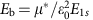, where E1s is the energy of the fundamental state of the hydrogen atom, μ* is the effective mass of electrons and holes (that is, 1/μ*=1/me+1/mh) and ε0 the static (electronic) dielectric constant of the solid. This model is very approximate but should provide a fair representation of the trends in exciton-binding energies in our compounds.

We calculated the effective mass tensors by approximating the second derivatives of the relativistic Kohn–Sham eigenvalues via finite differences, and taking the isotropic average in each case. Supplementary Fig. 2a,b shows the hole and electron effective masses thus obtained for the Platonic perovskite model, as a function of equatorial and apical metal–halide–metal bond angles. Our calculated effective masses are in line with previous calculations for CH3NH3PbI3 and CsPbI3 (refs 20, 21, 22).

For the dielectric constant we performed finite-electric field calculations using the Berry-phase technique60,61. In this case we adopted a Γ-centred 2 × 2 × 4 Brillouin-zone mesh, with a finite electric field of 0.001 a.u. directed along the c axis. While in this case we did not not evaluate the isotropic average (purely in order to contain computational costs), Supplementary Figure 2d shows that this should not pose a problem since ε0 does not depend strongly on the bond angles in the range of interest (ε0=5.5–6.0).

### Platonic model of metal–halide perovskites

Our ideal model of ABX3 metal–organic halide perovskites consists of four inequivalent BX6 Platonic octahedra in an orthorombic unit cell17, in absence of the cation. The octahedra are labelled O1–O4, as indicated in Fig. 1d, and are connected in a corner-sharing configuration. The B atoms occupy the centres of the octahedra, the X atoms are located at the corners and the metal–halide B–X distance is denoted by d. We set the origin of the reference frame at the centre of the octahedron O1, with its axes along the primitive vectors of the crystal lattice. The centres of the remaining three octahedra O2–O4 are denoted by (x,y,0), (0,0,z) and (x,y,z), respectively. The coordinates x, y and z will be determined below starting from the Euler angles described in the main text. The lattice parameters are expressed in terms of the centres of the octahedra as a=2x, b=2y and c=2z. The corners of the octhedra are labelled as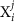, with the superscript j identifying the octahedron, and the subscript i identifying a corner of the octahedron, as shown in Fig. 1c. The coordinates of each cornerare referred to as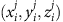.

Octahedron O1. We consider an initial configuration where the octahedron O1 has its apical diagonal along the c axis, and its equatorial edges along the a and b axes. The coordinates of the corners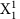,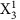and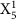are by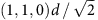,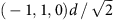and (0,0,1)d, respectively, while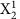,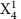and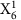are obtained from these by changing the sign of all coordinates. In order to generate the most general structural models we perform a rotation of O1 according to the three Euler angles, θ and φ. These angles define a sequence of spin, tilt and precession around the c axis. The tilt θ is performed via a rotation around a. This is illustrated in Fig. 1c. Following ref. 30 the matrix defining the rotation of the octahedron is:By applying this rotation to the initial coordinates of O1 we obtain the position of the corners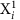(i=1,…,6) in their final orientation. These new coordinates are used to locate the centres and orientation of the remaining three octahedra.

Octahedron O2. In order to determine the centre (x, y, 0) of the octahedron O2 we use the following observations: O1 shares the corner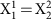with O2, and the corner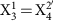with O2′, the periodic replica of O2 along the a axis. Furthermore, O2 shares the corner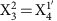with O1′, the periodic replica of O1 along the b axis. By requesting that the distance between the centre (Ci) of each octahedron and each corner is d in all cases, and that O2 and O2′ are both regular, we obtain the following equations relating the centre of O2 with the position of the corner: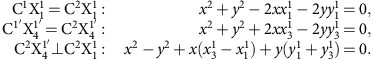After expressing the coordinates ofin terms of the Euler angles, θ, and φ, we find that the above relations are verified simultaneously only when the spinning angle vanishes,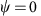. In this case the coordinates of the centre of O2 are given by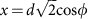,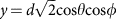and the coordinates of its corners are determined using the condition that the octahedron be regular. The explicit expressions for the coordinates of O2 in terms of the corners of O1 are: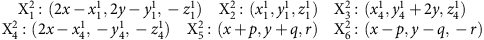where the auxiliary variables p, q, r are given by: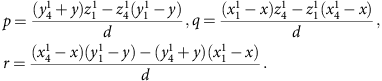From the location of O2 we can also determine the lattice parameters: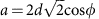,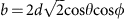and c=4d cosθ, where the last equation follows simply from the common tilt angle of O1 and O2 and the fact that they represent half of the unit cell along the c axis.

In the case of vanishing tilt angle, θ=0, the spinning angle and the precession angle identify the same rotation, hence their distinction is not meaningful. In this case the relevant parameter appearing in all the equations is their sum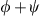. For consistency with the above rule thatwhenever θ≠0, we here adopt the convention thatalways. In this case, when θ=0 the only other independent parameter is the precession angle φ. Obviously, this choice does not affect our results in any way.

Octahedra O3 and O4. From Fig. 1d we see that octahedron O3 shares the corner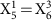with O1. As a consequence we have that octahedra O3 and O4 are centred at the positions 2d(0,0,cosθ) and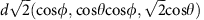, respectively. The corners of these octahedra are readily obtained by taking into account their regularity and the corner-sharing connectivity between O3 and O4 and between O2 and O4.

From the relations stated here it is clear that a cubic perovskite is obtained in the special case θ=φ=0. Furthermore, when the tilt angle vanishes, θ=0, we obtain two inequivalent tetragonal structures with identical lattice parameters. These structures correspond to the situations where the spinning angles of neighbouring octahedra along the apical direction are in-phase (same magnitude and same sign) or out-of-phase (same magnitude but different sign).

As a general remark, the fact that the structure of the Platonic model is completely defined once we specify the values of two angles and the B–X bond length is quite remarkable. This property simplifies considerably the task of rationalizing the physics of metal–organic halide perovskites. Furthermore, the model illustrated here carries general validity and it can easily be adapted to describe other idealized perovskite structures.

Apical and equatorial bond angles. The Euler angles θ and φ can be related to the intrinsic B–X–B bond angles. To this end we observe in Fig. 1d that αe is the angle between O1 and O2 through the corner, and αa is the angle between O1 and O3 through. Using the coordinates of the centres of O1–O3 and the cornersanddetermined as above, we find immediately cosαa=1−2cos2θ and cosαe=1−cos2φ(1+cos2θ). Since cos2(φ)≤1, the last two relations can be combined to yield the constraint cos(παa)≥2cos(παe)−1. For all the angles considered in Fig. 2, the expansion of this inequality to first order in the angles is accurate to within 3% and reads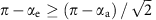. As a result, the Platonic model does not admit solutions with continuous corner-sharing connectivity in a region of the map bound by a straight line, as can be seen in Fig. 2.

### Tolerance factor

The Goldschmidt tolerance factor is given by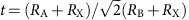, with RA, RB and RX the ionic radii of the elements in ABX3 perovskites42. It was found empirically that 3D perovskite form when t is in the range 0.7–1.1 (refs 33, 42, 43, 44). The lower bound corresponds to a situation where the cation is close enough to the halogen component to form a bond, thereby preventing the formation of a perovskite structure. The upper bound describes the case of the close-packed cubic perovskite structure. For values of t larger than 1 the perovskite structure is expected to distort or even form a layered perovskite structure62. For each structure we evaluated the Goldschmidt tolerance factor by using the steric sizes calculated via the DFT electron density and reported in Supplementary Table 1.

### Material synthesis and characterization

Perovskite precursor preparation. APbI3 precursors were prepared by dissolving equimolar amounts of AI (where A=Li, Na, K, Rb and Cs) and PbI2 in N,N-dimethylformamide at 0.5 M, in a nitrogen-filled glovebox. Mixtures were made by simply mixing the prepared precursors in the desired ratio. Film formation. Glass substrates were cleaned with acetone, isopropanol and oxygen plasma treatment. Thin films were formed by spin-coating on the substrates at 2,000 r.p.m. in the glovebox, and subsequently annealed on a hotplate by increasing the temperature until the formation of the dark phase was observed. This occurred at ~400 °C for CsPbI3 and even higher for the Cs/Rb mixtures discussed in the main text. The 11% Rb film changed colour at 420 °C and the 20% Rb film at 470 °C. Optical measurements. Absorbance spectra were collected with a Varian Cary 300 UV–vis spectrophotometer with an internally coupled integrating sphere.